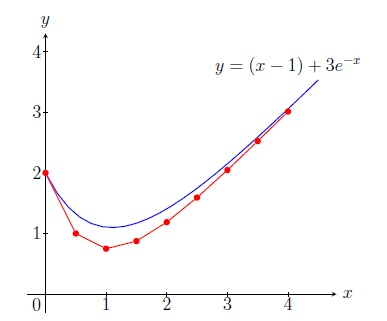Calculus II Home

## Euler's Method

Can we numerically find an approximate solution to the following ODE? $\begin{equation*} \displaystyle\frac{dy}{dx}=f(x,y),\, y(x_0)=y_0\\ \end{equation*}$

Euler's Method:
The tangent line to a solution $$y=\phi(x)$$ at the point $$(x_0,y_0)$$ has slope $$\displaystyle\left.\frac{dy}{dx}\right]_{(x_0,y_0)}=f(x_0,y_0)$$. So an equation of the tangent line would be $y=y_0+(x-x_0)f(x_0,y_0).$ If $$x_1$$ is close to $$x_0$$, then $$y_1=y_0+(x_1-x_0)f(x_0,y_0)$$ would be a good approximation to $$y=\phi(x_1)$$. Similarly if $$x_2$$ is close to $$x_1$$, then $$y_2=y_1+(x_2-x_1)f(x_1,y_1)$$ would be a good approximation to $$y=\phi(x_2)$$. Thus a general formula for this tangent line expression would be $\begin{equation} y_{n+1}=y_n+(x_{n+1}-x_n)f(x_n,y_n),\,n=0,1,2,\ldots.\;\;\;\;\;\;\;\; (1) \end{equation}$ If we take $$x_0,x_1,x_3,\ldots$$ with a fixed difference $$h$$ (i.e. $$x_{n+1}=x_n+h$$), then (1) becomes $\begin{equation} \boxed{y_{n+1}=y_n+hf(x_n,y_n),\, n=0,1,2,\ldots.}\;\;\;\;\;\;\;\; (2)\\ \end{equation}$

Example. Use Euler's method with step size $$h=0.5$$ to approximate $$y(4)$$ where $$y=\phi(x)$$ is a solution to the following ODE. $\frac{dy}{dx}=x-y,\, y(0)=2$ Solution. We have $$h=0.5,\, x_0=0,\, y_0=2$$ and $$f(x,y)=x-y$$. So from (2) we have $y_{n+1}=y_n+0.5(x_n-y_n),\, n=0,1,2,\ldots.$ \begin{align*} x_1&=x_0+0.5=0.5, &&y_1=y_0+0.5(x_0-y_0)=2+0.5(0-2)=1\\ x_2&=x_1+0.5=1, &&y_2=y_1+0.5(x_1-y_1)=1+0.5(0.5-1)=0.75\; etc. \end{align*} $\begin{array}{|c|l|l|} \hline n& x_n & y_n \\ \hline 0&0 &2\\ \hline 1&0.5 &1\\ \hline 2&1 &0.75\\ \hline 3&1.5 &0.875\\ \hline 4&2 &1.1875\\ \hline 5&2.5 &1.59375\\ \hline 6&3 &2.046875\\ \hline 7&3.5 &2.5234375\\ \hline 8&4 &3.01171875\\ \hline \end{array}$ So $$y(4) \approx y_8=3.01171875$$, i.e., $$y(4)$$ is approximately $$y_8=3.01171875$$.Euler's tangent line approximation to $$\frac{dy}{dx}=x-y,\, y(0)=2$$ with $$h=0.5$$

Checking solution: Solving $$\displaystyle\frac{dy}{dx}=x-y,\, y(0)=2$$, we get $$y=(x-1)+3e^{-x}$$. The exact value of $$y(4)$$ is $$(4-1)+3e^{-4}=3.0183$$ which was approximated by $$3.0117$$. (pretty close!)

Last edited